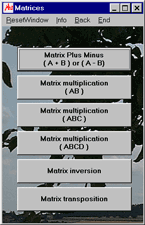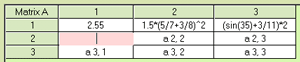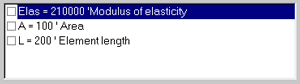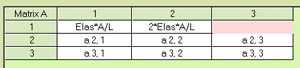# MasterAllRound: the PC calculator

## Matrices#### Matrix operations

MasterAllRound offers several matrix operations. There is no size restriction for MasterAllRound:
• Matrix addition and matrix subtraction
• Matrix multiplication A · B
• Matrix multiplication A · B · C
• Matrix multiplication A · B · C · D
• Matrix inversion
• Matrix transposition

#### Plain text input

As with the universal calculator, the matrix modules of MasterAllRound interpret your plain text input. You have several options to edit the cells:
•You can enter numbers or formulas directly into the cells. For example:
• 2.55
• 1.5 * (5/7 + 3/8)^2
• (sin(35) + 3/11)*2

•Alternatively, you can work with number symbols. In this case, you assign values to the constants, first using the smart calculator (the "smart" version of the universal calculator). As in the universal calculator you can enter comments, for example:
• Elas = 210000 'Modulus of elasticity
• A = 100 'Area
• L = 200 'Element lengthIn a second step, you make entries to the matrix cells. For example:
• Elas*A/L
• 2*Elas*A/L

#### Application examples

Matrix computations are widely used, e. g. in structural engineering and economics: